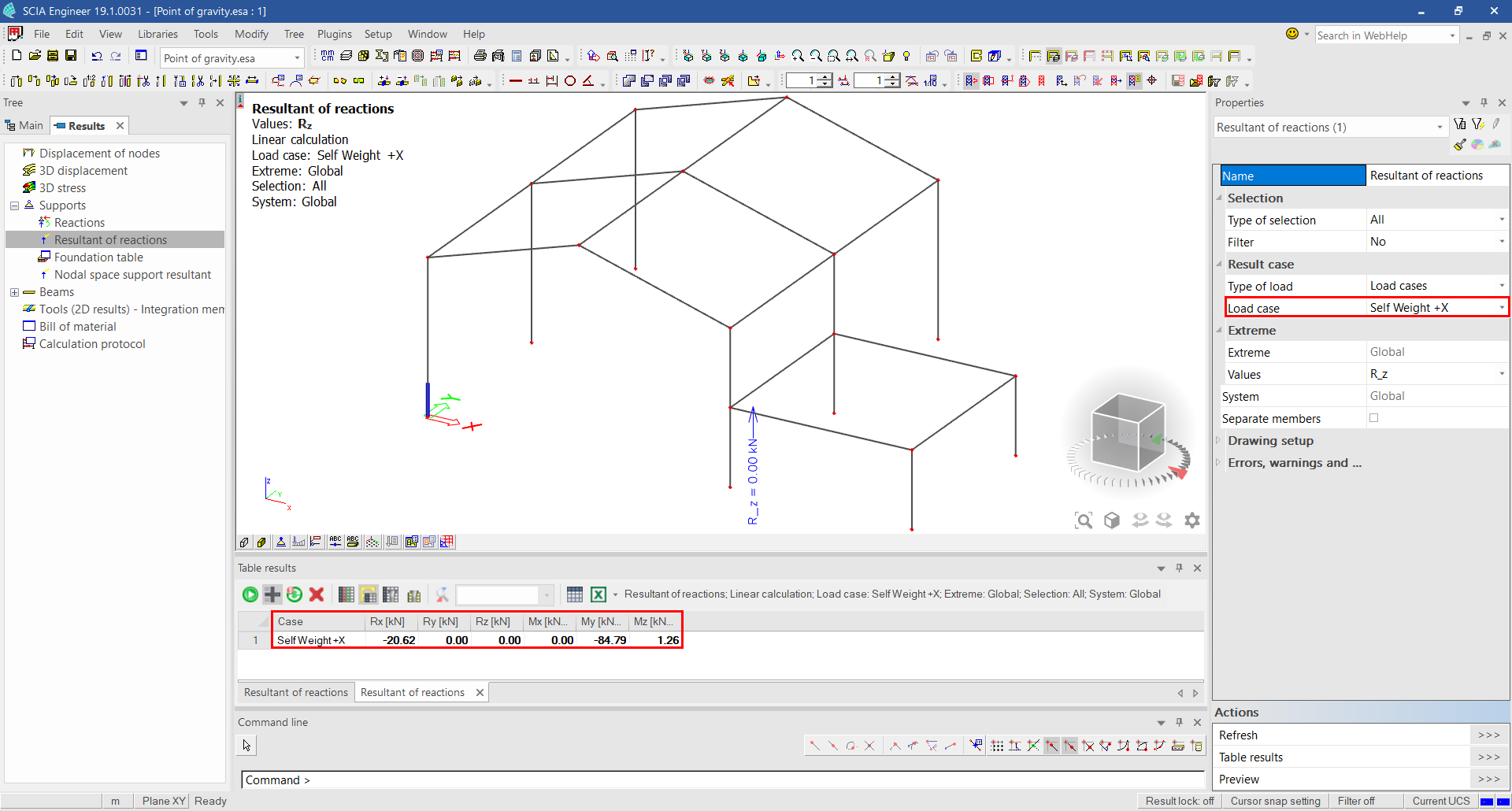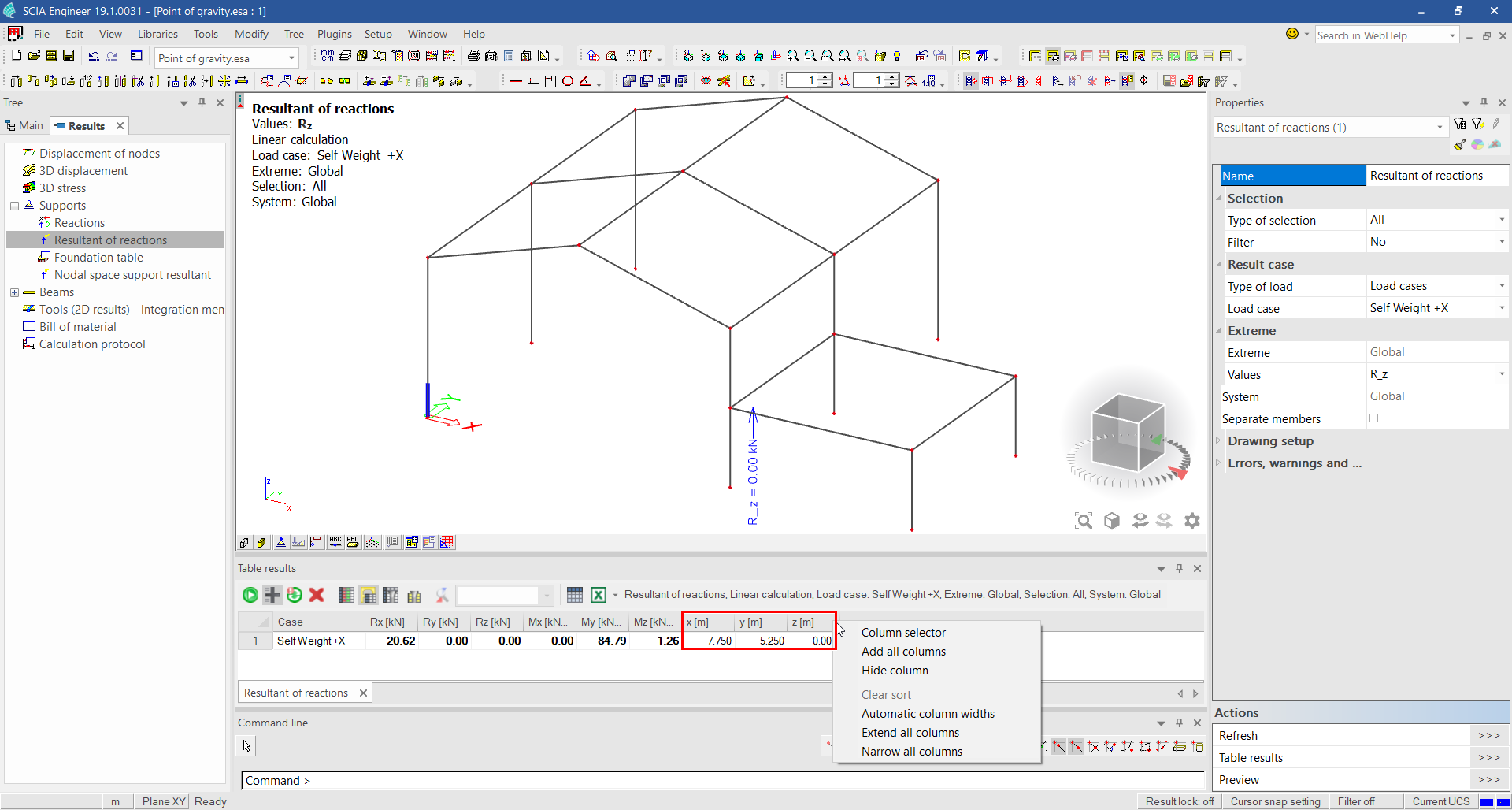# How can the center of gravity (mass) be calculated?

The only possibility to calculate the center of gravity is by means of the resultant of reactions & the position of the central point.

Then the resultant internal forces can be calculated back to find the center of gravity.

### STEP 1: Create 3 Load cases

Create 3 load cases: 'Self Weight +X', 'Self Weight +Y' and 'Self Weight +Z'

The figure below shows the settings for load case 'Self Weight +X'. The other load cases are set similarly.

### STEP 2: CALCULATION

Execute the linear calculation.

### STEP 3: RESULTS

Look at the result 'Resultant of reactions' for the 3 created load cases.

The figure below shows the result for load case 'Self Weight +X'You can copy these table results immediately to Excel.

You also need the central point. The coordinates of this point can be found as well in the table results (if this is not visible, you can right-click in the bar and choose for 'Add all columns').### STEP 4: calculate the CENTER OF GRAVITY

The equations for a 3D structure in the center of gravity are:

Mx = Rz*dY - Ry*dZ; My = Rx*dZ - Rz*dX and Mz = Ry*dX - Rx*dY

For load case 'Self Weight +X' (Ry and Rz are 0) this becomes:

My = Rx*dZ => dZ = My/Rx and Mz = -Rx*dY => dY = -Mz/Rx

For 'Self Weight +Y' (Rx and Rz are 0) this becomes:

Mx = -Ry*dZ => dZ = -Mx/Ry and Mz = Ry*dX => dX = Mz/Ry

For 'Self Weight +Z' (Rx and Ry are 0) this becomes:

Mx = Rz*dY => dY = Mx/Rz and My = -Rz*dX => dX = -My/Rz

Calculate the center of gravity (Xc, Yc, Zc) with: Xc = X + dXYc = Y + dY and Zc = Z + dZ

(X, Y, Z) are the coordinates of the central point.

Following Excel-file calculates the point of gravity automatically with the formulas that are described above:calculate_center_of_gravity.xlsx